# QUOTIENT function

Returns one number divided by another, without the remainder.

### Sample Usage

`QUOTIENT(4,2)`

`QUOTIENT(A2,B2)`

### Syntax

`QUOTIENT(dividend, divisor)`

• `dividend` - The number to be divided.

• `divisor` - The number to divide by (cannot equal `0`).

### Notes

• `QUOTIENT` performs a division, but will only return the quotient and not the remainder. To see the full result (quotient and remainder), use the `DIVIDE` function or the '/' operator.

`SUM`: Returns the sum of a series of numbers and/or cells.

`PRODUCT`: Returns the result of multiplying a series of numbers together.

`MULTIPLY`: Returns the product of two numbers. Equivalent to the `*` operator.

`MINUS`: Returns the difference of two numbers. Equivalent to the `-` operator.

`DIVIDE`: Returns one number divided by another. Equivalent to the `/` operator.

`ADD`: Returns the sum of two numbers. Equivalent to the `+` operator.

# QUOTIENT function

Returns one number divided by another, without the remainder.

### Sample Usage

`QUOTIENT(4,2)`

`QUOTIENT(A2,B2)`

### Syntax

`QUOTIENT(dividend, divisor)`

• `dividend` - The number to be divided.

• `divisor` - The number to divide by (cannot equal `0`).

### Notes

• `QUOTIENT` performs a division, but will only return the quotient and not the remainder. To see the full result (quotient and remainder), use the `DIVIDE` function or the '/' operator.

`SUM`: Returns the sum of a series of numbers and/or cells.

`PRODUCT`: Returns the result of multiplying a series of numbers together.

`MULTIPLY`: Returns the product of two numbers. Equivalent to the `*` operator.

`MINUS`: Returns the difference of two numbers. Equivalent to the `-` operator.

`DIVIDE`: Returns one number divided by another. Equivalent to the `/` operator.

`ADD`: Returns the sum of two numbers. Equivalent to the `+` operator.

# QUOTIENT function

Returns one number divided by another, without the remainder.

### Sample Usage

`QUOTIENT(4,2)`

`QUOTIENT(A2,B2)`

### Syntax

`QUOTIENT(dividend, divisor)`

• `dividend` - The number to be divided.

• `divisor` - The number to divide by (cannot equal `0`).

### Notes

• `QUOTIENT` performs a division, but will only return the quotient and not the remainder. To see the full result (quotient and remainder), use the `DIVIDE` function or the '/' operator.

`SUM`: Returns the sum of a series of numbers and/or cells.

`PRODUCT`: Returns the result of multiplying a series of numbers together.

`MULTIPLY`: Returns the product of two numbers. Equivalent to the `*` operator.

`MINUS`: Returns the difference of two numbers. Equivalent to the `-` operator.

`DIVIDE`: Returns one number divided by another. Equivalent to the `/` operator.

`ADD`: Returns the sum of two numbers. Equivalent to the `+` operator.

# QUOTIENT function

Returns one number divided by another, without the remainder.

### Sample Usage

`QUOTIENT(4,2)`

`QUOTIENT(A2,B2)`

### Syntax

`QUOTIENT(dividend, divisor)`

• `dividend` - The number to be divided.

• `divisor` - The number to divide by (cannot equal `0`).

### Notes

• `QUOTIENT` performs a division, but will only return the quotient and not the remainder. To see the full result (quotient and remainder), use the `DIVIDE` function or the '/' operator.

`SUM`: Returns the sum of a series of numbers and/or cells.

`PRODUCT`: Returns the result of multiplying a series of numbers together.

`MULTIPLY`: Returns the product of two numbers. Equivalent to the `*` operator.

`MINUS`: Returns the difference of two numbers. Equivalent to the `-` operator.

`DIVIDE`: Returns one number divided by another. Equivalent to the `/` operator.

`ADD`: Returns the sum of two numbers. Equivalent to the `+` operator.

This article has been archived and is no longer updated by Apple.

Keeping your software up to date is essential to maintaining the security of your Mac. Updates also offer new features and can improve stability, performance, and compatibility. And when updates download automatically, it's faster and more convenient to install them when you're ready.

If you're a system administrator and need to prevent automatic download of software updates, such as to limit data usage or conserve network bandwidth, you can use any of these solutions.

## Use Software Update preferences

Use Software Update preferences to turn automatic software downloads on or off. This method is available to anyone administering their personal Mac computer.

## Use a configuration profile

System administrators can prevent automatic downloads by using a configuration profile with the `AutomaticDownload` key set to `False` in the com.apple.SoftwareUpdate domain.

## Use a Terminal command

`  sudo defaults write /Library/Preferences/com.apple.SoftwareUpdate AutomaticDownload -boolean FALSE  `

Published Date:

# QUOTIENT function

Returns one number divided by another, without the remainder.

### Sample Usage

`QUOTIENT(4,2)`

`QUOTIENT(A2,B2)`

### Syntax

`QUOTIENT(dividend, divisor)`

• `dividend` - The number to be divided.

• `divisor` - The number to divide by (cannot equal `0`).

### Notes

• `QUOTIENT` performs a division, but will only return the quotient and not the remainder. To see the full result (quotient and remainder), use the `DIVIDE` function or the '/' operator.

`SUM`: Returns the sum of a series of numbers and/or cells.

`PRODUCT`: Returns the result of multiplying a series of numbers together.

`MULTIPLY`: Returns the product of two numbers. Equivalent to the `*` operator.

`MINUS`: Returns the difference of two numbers. Equivalent to the `-` operator.

`DIVIDE`: Returns one number divided by another. Equivalent to the `/` operator.

`ADD`: Returns the sum of two numbers. Equivalent to the `+` operator.

# QUOTIENT function

Returns one number divided by another, without the remainder.

### Sample Usage

`QUOTIENT(4,2)`

`QUOTIENT(A2,B2)`

### Syntax

`QUOTIENT(dividend, divisor)`

• `dividend` - The number to be divided.

• `divisor` - The number to divide by (cannot equal `0`).

### Notes

• `QUOTIENT` performs a division, but will only return the quotient and not the remainder. To see the full result (quotient and remainder), use the `DIVIDE` function or the '/' operator.

`SUM`: Returns the sum of a series of numbers and/or cells.

`PRODUCT`: Returns the result of multiplying a series of numbers together.

`MULTIPLY`: Returns the product of two numbers. Equivalent to the `*` operator.

`MINUS`: Returns the difference of two numbers. Equivalent to the `-` operator.

`DIVIDE`: Returns one number divided by another. Equivalent to the `/` operator.

`ADD`: Returns the sum of two numbers. Equivalent to the `+` operator.

# QUOTIENT function

Returns one number divided by another, without the remainder.

### Sample Usage

`QUOTIENT(4,2)`

`QUOTIENT(A2,B2)`

### Syntax

`QUOTIENT(dividend, divisor)`

• `dividend` - The number to be divided.

• `divisor` - The number to divide by (cannot equal `0`).

### Notes

• `QUOTIENT` performs a division, but will only return the quotient and not the remainder. To see the full result (quotient and remainder), use the `DIVIDE` function or the '/' operator.

`SUM`: Returns the sum of a series of numbers and/or cells.

`PRODUCT`: Returns the result of multiplying a series of numbers together.

`MULTIPLY`: Returns the product of two numbers. Equivalent to the `*` operator.

`MINUS`: Returns the difference of two numbers. Equivalent to the `-` operator.

`DIVIDE`: Returns one number divided by another. Equivalent to the `/` operator.

`ADD`: Returns the sum of two numbers. Equivalent to the `+` operator.

# POWER function

Returns a number raised to a power.

### Sample Usage

`POWER(4,0.5)`

`POWER(A2,B2)`

`POWER(2,5)`

### Syntax

`POWER(base, exponent)`

• `base` - The number to raise to the `exponent` power.

• If `base` is negative, `exponent` must be an integer.
• `exponent` - The exponent to raise `base` to.

`SQRTPI`: Returns the positive square root of the product of Pi and the given positive number.

`SQRT`: Returns the positive square root of a positive number.

`LOG10`: Returns the the logarithm of a number, base 10.

`LOG`: Returns the the logarithm of a number given a base.

`LN`: Returns the the logarithm of a number, base e (Euler's number).

`GAMMALN`: Returns the the logarithm of a specified Gamma function, base e (Euler's number).

`EXP`: Returns Euler's number, e (~2.718) raised to a power.

### Leave a group text message on your iPhone, iPad, or iPod touch - Apple Support

Leave a group text message on your iPhone, iPad, or iPod touch

You can leave a group text message as long as there are three other people on the thread and everyone is using an Apple device, like an iPhone, iPad, or iPod touch. You can also mute notifications for certain group text messages.

To leave a group text, everyone needs to be using iMessage. Not sure if you're using iMessage or SMS/MMS? Learn the difference between iMessage and SMS/MMS.## How to leave a group text message

1. Tap the group text message that you want to leave.
2. Tap the group icons at the top of the thread.
3. Tap the info button, scroll down, then tap Leave this Conversation.

You can leave a group text message as long as there are three other people on the thread and everyone is using an Apple device, like an iPhone, iPad, or iPod touch. If you don't see the option to leave, it might mean that one or more of the users isn't using an Apple device with iMessage. If you can't leave a group text message, you can mute the conversation so you don't get notifications.## How to mute a group text message

1. Tap the group text message.
2. Tap the group icons at the top of the thread, then tap the info button.
3. Scroll down, then turn on Hide Alerts.

Or, from your list of messages conversations, you can swipe left over the group text message and tap the Alerts button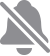. When Hide Alerts is on, a crescent moon icon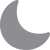appears next to the conversation.Published Date:

# PI

The PI function returns the value of pi to 9 decimal places.

`PI()`

### Syntax

`PI()`

`TANH`: The TANH function returns the hyperbolic tangent of any real number.

`TAN`: The TAN function returns the tangent of an angle provided in radians.

`SINH`: The SINH function returns the hyperbolic sine of any real number.

`SIN`: The SIN function returns the sine of an angle provided in radians.

`RADIANS`: The RADIANS function converts an angle value in degrees to radians.

`DEGREES`: The DEGREES function converts an angle value in radians to degrees.

`COSH`: The COSH function returns the hyperbolic cosine of any real number.

`COS`: The COS function returns the cosine of an angle provided in radians.

`ATANH`: The ATANH function returns the inverse hyperbolic tangent of a number.

`ATAN2`: The ATAN2 function returns the angle between the x-axis and a line segment from the origin (0,0) to the specified coordinate pair (`x`,`y`), in radians.

`ATAN`: The ATAN function returns the inverse tangent of a value in radians.

`ASINH`: The ASINH function returns the inverse hyperbolic sine of a number.

`ASIN`: The ASIN function returns the inverse sine of a value in radians.

`ACOSH`: The ACOSH function returns the inverse hyperbolic cosine of a number.

`ACOS`: The ACOS function returns the inverse cosine of a value in radians.

# MULTINOMIAL

Returns the factorial of the sum of values divided by the product of the values' factorials.

### Sample Usage

`MULTINOMIAL(1,2,3)`

`MULTINOMIAL(A2:A9)`

### Syntax

`MULTINOMIAL(value1, [value2, ...])`

• `value1` - The first value or range to consider.

• `value2, ...` - Additional values or ranges to consider.

### Notes

• Although `MULTINOMIAL` is specified as taking a maximum of 30 arguments, Google Sheets supports an arbitrary number of arguments for this function.

`FACTDOUBLE`: Returns the "double factorial" of a number.

`FACT`: The FACT function returns the factorial of a number.

`COMBIN`: The COMBIN function returns the number of ways to choose some number of objects from a pool of a given size of objects.

# MROUND

Rounds one number to the nearest integer multiple of another.

### Sample Usage

`MROUND(21,14)`

`MROUND(A2,3)`

### Syntax

`MROUND(value,factor)`

• `value` - The number to round to the nearest integer multiple of another.

• `factor` - The number to whose multiples `value` will be rounded.

### Notes

• Both `value` and `factor` may be non-integral.

• `value` and `factor` must have the same sign; that is, they must both be positive or both negative. If either is zero, `MROUND` will return `0`.

• If `value` is equally close to two multiples of `factor`, the multiple with the greater absolute value will be returned.

`TRUNC`: Truncates a number to a certain number of significant digits by omitting less significant digits.

`ROUNDUP`: Rounds a number to a certain number of decimal places, always rounding up to the next valid increment.

`ROUNDDOWN`: The ROUNDDOWN function rounds a number to a certain number of decimal places, always rounding down to the next valid increment.

`ROUND`: The ROUND function rounds a number to a certain number of decimal places according to standard rules.

`INT`: Rounds a number down to the nearest integer that is less than or equal to it.

`FLOOR`: The FLOOR function rounds a number down to the nearest integer multiple of specified significance.

`CEILING`: The CEILING function rounds a number up to the nearest integer multiple of specified significance.

# LOG10

Returns the the logarithm of a number, base 10.

### Sample Usage

`LOG10(100)`

`LOG10(A2)`

### Syntax

`LOG10(value)`

• `value` - The value for which to calculate the logarithm, base 10.

• `value` must be positive.

### Notes

• Google Sheets does not support complex or imaginary values.

• `LOG10` is equivalent to `LOG` given `base` of 10.

`SQRTPI`: Returns the positive square root of the product of Pi and the given positive number.

`SQRT`: Returns the positive square root of a positive number.

`POWER`: Returns a number raised to a power.

`LOG`: Returns the the logarithm of a number given a base.

`LN`: Returns the the logarithm of a number, base e (Euler's number).

`GAMMALN`: Returns the the logarithm of a specified Gamma function, base e (Euler's number).

`EXP`: Returns Euler's number, e (~2.718) raised to a power.

### Examples

Learn how to view and manage your notifications to minimize interruptions throughout the day.## Open from the Lock screen

You can see your recent notifications on the Lock screen by picking up your iPhone or waking your iPad. You can also do these things from the Lock screen:

• Tap a single notification to open the app that it's from.
• Tap a group of notifications to view all recent notifications from that app.
• Touch and hold a notification* to view the notification and perform quick actions if the app offers them.

To show the contents of notifications on the Lock screen without unlocking your device, go to Settings > Notifications > Show Previews, and select Always.

* On iPhone SE (2nd generation), swipe left on the notification, then tap View to see the notification and perform any quick actions that the app supports.• From the Lock screen, swipe up from the middle of the screen.
• From any other screen, swipe down from the center of the top of your screen.

To clear your notification history, touch and hold the X button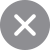and tap Clear All Notifications. Or tap the X buttonand tap Clear.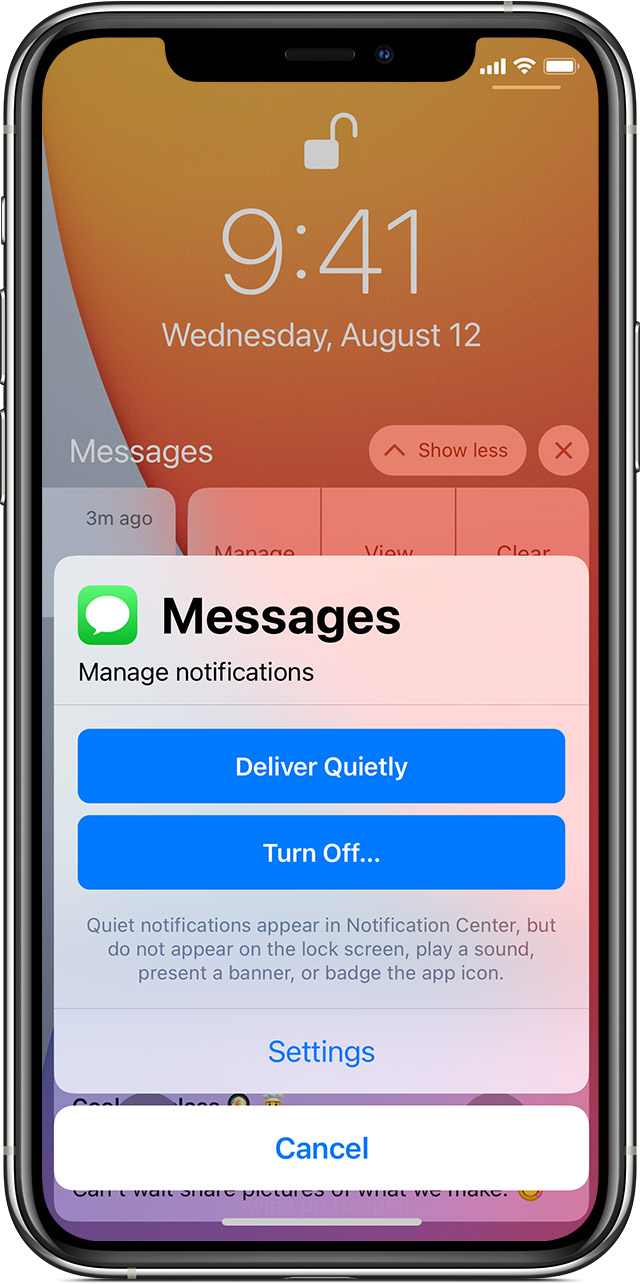2. Tap Manage, then select from these options:
• Deliver Quietly: These notifications appear in the Notification Center, but don't show up on the Lock screen, play sounds, or show a banner or badge icon.
• Turn Off: This turns off all notifications for a specific app. To turn this back on, go to Settings > Notifications, select the app, and tap Allow Notifications.

You can also tap Settings to change your alert styles or alert settings for a specific app.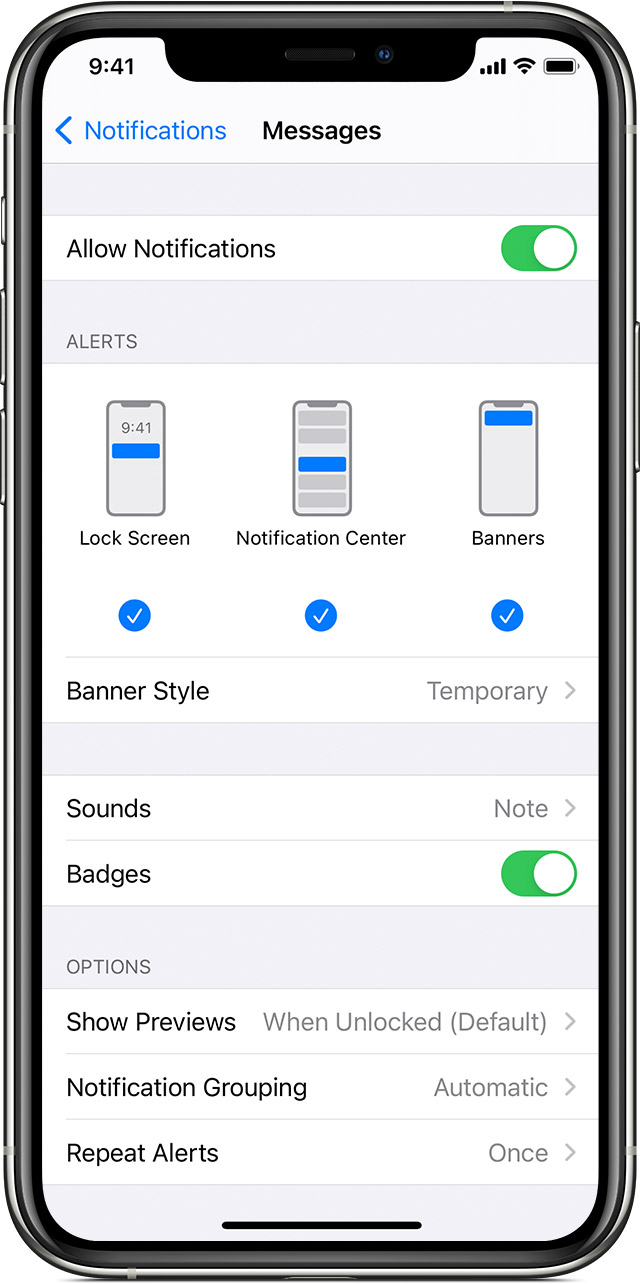1. Go to Settings > Notifications.
2. Select an app under Notification Style.
3. Choose a type of alert and banner style.

You can also turn sounds and badges on or off.

You can use group notifications or keep them separate.

1. Go to Settings > Notifications.
2. Select an app and tap Notification Grouping.
3. Select one of these options:
• Automatic: Notifications from each app will appear in groups based on app alerts.
• By App: All notifications from each app will be grouped into single expandable alerts.

From here you can also choose how to show notification previews for specific apps. For example, you can have notification previews show up all the time or never, even when your device is locked.Some apps use your location to send you relevant alerts based on where you are. For example, you might get a reminder to call someone when you get to a specific place or when you leave for your next location.

If you don't want these type of alerts, you can turn them off. Go to Settings > Privacy > Location Services, then tap an app to turn alerts on or off based on your location. Learn more about Location Services.## If you don't see notifications for a specific app

To get notifications, connect to a Wi-Fi or cellular network. If you still don't get notifications for a specific app, try these steps:

1. Make sure that the app supports notifications. Go to Settings > Notifications, select the app, and make sure that Allow Notifications is on.
2. If you have notifications turned on for an app but you're not receiving alerts, you might not have Banners selected. Go to Settings > Notifications, select the app, then select Banners.
3. Make sure that you're signed in to your Apple ID
4. Make sure that Do Not Disturb is off
5. If you've recently installed an app or restored from a backup, open the app to start getting notifications.Notifications use Wi-Fi only when a cellular connection is unavailable. Firewalls and proxy servers might affect your ability to receive notifications. Get help using Apple Push Notification service (APNs)

Information about products not manufactured by Apple, or independent websites not controlled or tested by Apple, is provided without recommendation or endorsement. Apple assumes no responsibility with regard to the selection, performance, or use of third-party websites or products. Apple makes no representations regarding third-party website accuracy or reliability. Contact the vendor for additional information.

Published Date:

# LN

Returns the the logarithm of a number, base e (Euler's number).

### Sample Usage

`LN(100)`

`LN(A2)`

### Syntax

`LN(value)`

• `value` - The value for which to calculate the logarithm, base e.

• `value` must be positive.

### Notes

• `LN` is equivalent to `LOG` given `base` of e, or `EXP(1)`.

`SQRTPI`: Returns the positive square root of the product of Pi and the given positive number.

`SQRT`: Returns the positive square root of a positive number.

`POWER`: Returns a number raised to a power.

`LOG10`: Returns the the logarithm of a number, base 10.

`LOG`: Returns the the logarithm of a number given a base.

`GAMMALN`: Returns the the logarithm of a specified Gamma function, base e (Euler's number).

`EXP`: Returns Euler's number, e (~2.718) raised to a power.

# LCM

Returns the least common multiple of one or more integers.

### Sample Usage

`LCM(A2:A5)`

`LCM(2,3,A4)`

### Syntax

`LCM(value1, [value2, ...])`

• `value1` - The first value or range whose factors to consider in a calculation to find the least common multiple.

• `value2, ...` - [ OPTIONAL ] - Additional values or ranges whose factors to consider to find the least common multiple.

### Notes

• Any input with a decimal part provided to `LCM` will be silently truncated.

`GCD`: Returns the greatest common divisor of one or more integers.

# ISEVEN

Checks whether the provided value is even.

### Sample Usage

`ISEVEN(4)`

`ISEVEN(A2)`

### Syntax

`ISEVEN(value)`

• `value` - The value to be verified as even.

• `ISEVEN` returns `TRUE` if `value` is an even integer or a reference to a cell containing an even integer, and `FALSE` otherwise.

### Notes

• This function is most often used in conjunction with `IF` in conditional statements.

`ISTEXT`: Checks whether a value is text.

`ISREF`: Checks whether a value is a valid cell reference.

`ISODD`: Checks whether the provided value is odd.

`ISNUMBER`: Checks whether a value is a number.

`ISNONTEXT`: Checks whether a value is non-textual.

`ISNA`: Checks whether a value is the error `#N/A`.

`ISLOGICAL`: Checks whether a value is `TRUE` or `FALSE`.

`ISERROR`: Checks whether a value is an error.

`ISERR`: Checks whether a value is an error other than `#N/A`.

`ISBLANK`: Checks whether the referenced cell is empty.

`IF`: Returns one value if a logical expression is `TRUE` and another if it is `FALSE`.

# GAMMALN

Returns the the logarithm of a specified Gamma function, base e (Euler's number).

### Sample Usage

`GAMMALN(4)`

`GAMMALN(A2)`

### Syntax

`GAMMALN(value)`

• `value` - The input to the Gamma function. The natural logarithm of Gamma (`value`) will be returned.

• `value` must be positive.

### Notes

• `GAMMALN` is used for certain types of analysis or as a component to other functions and is rarely used by itself.

`SQRTPI`: Returns the positive square root of the product of Pi and the given positive number.

`SQRT`: Returns the positive square root of a positive number.

`POWER`: Returns a number raised to a power.

`LOG10`: Returns the the logarithm of a number, base 10.

`LOG`: Returns the the logarithm of a number given a base.

`LN`: Returns the the logarithm of a number, base e (Euler's number).

`EXP`: Returns Euler's number, e (~2.718) raised to a power.

### How to delete messages in iCloud - Apple Support

How to delete messages in iCloud

With Messages in iCloud, your entire message history stays up to date across your devices. If you need to free up space in your iCloud storage, you can delete individual messages from all of your devices or turn off the Messages in iCloud feature.

## What happens when you delete messages or turn off the Messages in iCloud feature

With Messages in iCloud, when you delete a message, attachment, or conversation on one device, it deletes from all of your devices. This can help free up some space in your iCloud storage, while keeping the rest of your conversations up to date across your devices. Once you delete a message, you can't get it back.

If you don't need to keep your conversations up to date across your devices, you can turn off the Messages in iCloud feature. If you turn off Messages in iCloud on an iPhone or iPad, your message history is included in a separate iCloud backup.## How to delete messages from iCloud

With Messages in iCloud enabled, if you delete a message in one place, it deletes everywhere.

To delete an individual message or attachment, follow these steps:

1. In a message conversation, touch and hold the message bubble or attachment that you want to delete.
2. Tap More.
3. Tap Trash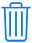, then tap Delete Message. If you want to delete all messages in the thread tap Delete All, in the upper-left corner. Then tap Delete Conversation.

To delete multiple attachments from a contact or conversation, follow these steps:

1. In a message conversation, tap the contact's name at the top of the screen.
2. Tap Info.
3. Select See All Photos
4. Tap Select, tap the images you'd like to delete.
5. Tap Delete, then tap Delete Attachment to confirm.

To delete an entire conversation, follow these steps:

1. Swipe left over the conversation that you want to delete.
2. Tap Delete.
3. Tap Delete again to confirm.

To delete an individual message or attachment, follow these steps:

1. Open a message conversation.
2. Control-click the blank area of a message bubble that you want to delete.
3. Choose Delete.
4. Click Delete.

To delete an entire conversation, follow these steps:

1. Control-click a conversation.
2. Choose Delete Conversation.
3. Click Delete.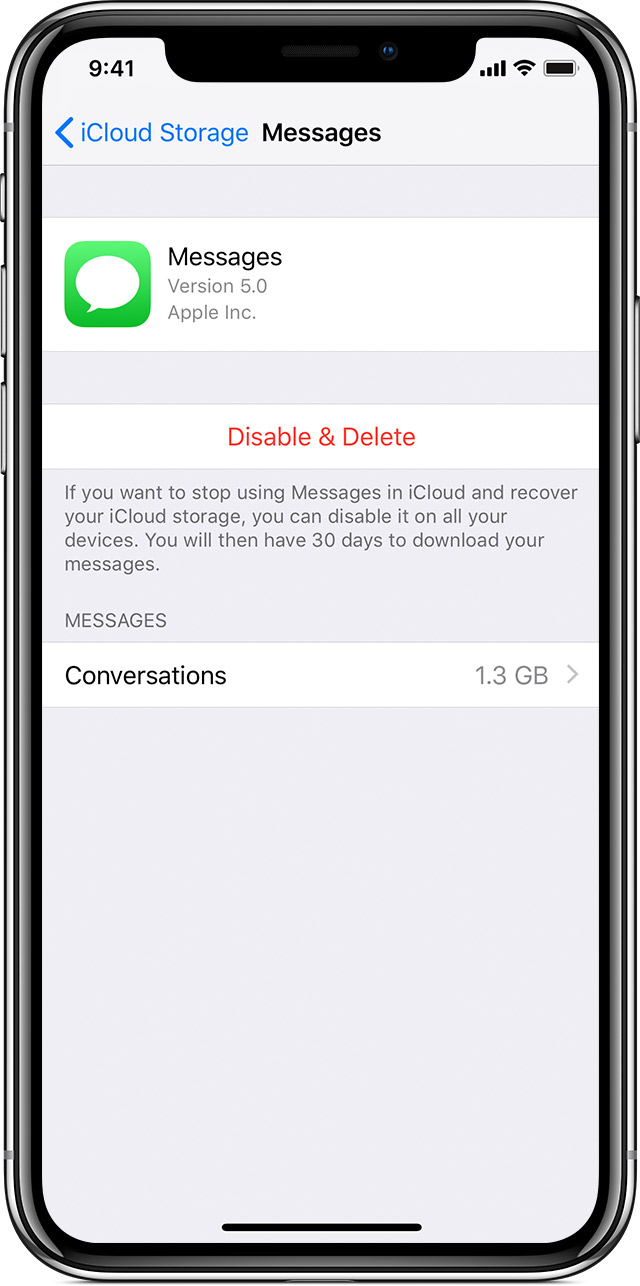## Turn off the Messages in iCloud feature

If you don't want to store your message history in iCloud and keep your conversations up to date across your devices, use the steps below to turn off Messages in iCloud.

1. Tap Settings > [your name].
2. Tap iCloud.
3. Tap Manage Storage.
4. Tap Messages, then tap Disable & Delete.

1. Open Messages, then choose Messages in the menu bar.
2. Select Preferences, then click iMessage.
3. Deselect Enable Messages in iCloud, then select Disable All.## If you can't delete messages on your Mac

If you delete individual messages from iCloud but you still see them on your Mac, you might need to edit your Messages settings on your Mac. In Messages, go to Preferences > General. Make sure that the box next to "Save history when conversations are closed" is deselected.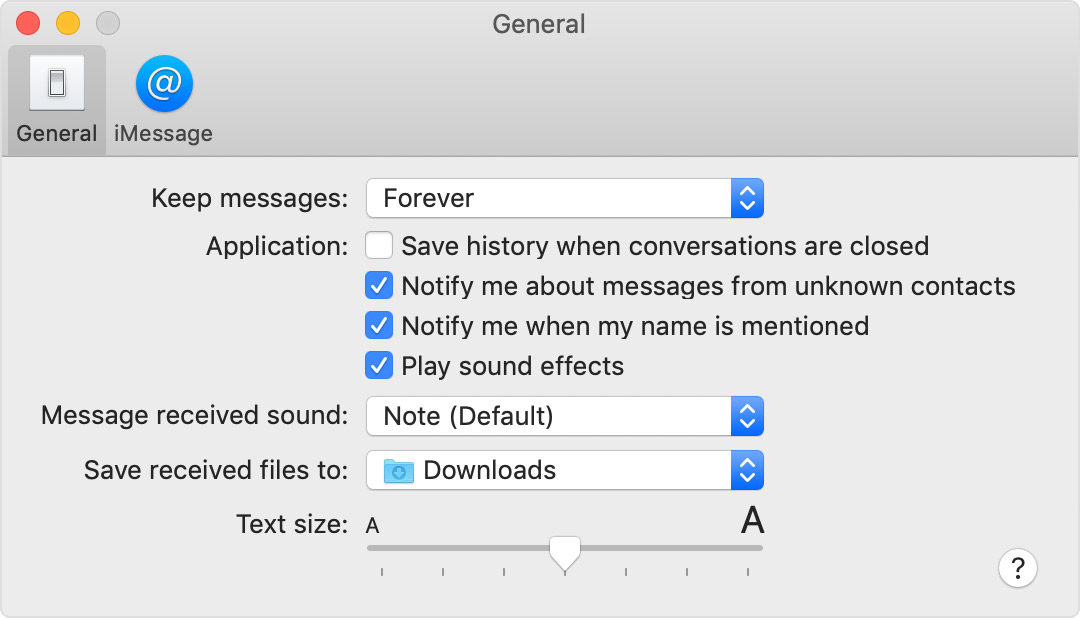When you go over your iCloud storage limit, your messages and attachments don't upload to iCloud, and your devices won't back up or download to iCloud. You can still access your most recent messages, but they won't be stored in iCloud and deleted messages will not update on all of your devices. To keep using Messages in iCloud, upgrade your storage or free up space by deleting files that you don't need.

Published Date:

# FACTDOUBLE

Returns the "double factorial" of a number.

### Sample Usage

`FACTDOUBLE(6)`

`FACTDOUBLE(A2)`

### Syntax

`FACTDOUBLE(value)`

• `value` - The number or reference to a number whose double factorial will be calculated and returned.

### Notes

• The double factorial is similar to the factorial, except instead of multiplying by each integer value less than or equal to the provided value, it decrements by 2. Thus the double factorial of 8 is 384 and the double factorial of 7 is 105.

• If a number or reference to a number with a decimal part is provided to `FACTDOUBLE`, the decimal part will be silently truncated before calculation.

`MULTINOMIAL`: Returns the factorial of the sum of values divided by the product of the values' factorials.

`FACT`: The FACT function returns the factorial of a number.

`COMBIN`: The COMBIN function returns the number of ways to choose some number of objects from a pool of a given size of objects.

# FACT

The FACT function returns the factorial of a number.

### Sample Usage

`FACT(3)`

`FACT(A2)`

### Syntax

`FACT(value)`

• `value` - The number or reference to a number whose factorial will be calculated and returned.

### Notes

• If a number or reference to a number with a decimal part is provided to `FACT`, the decimal part will be silently truncated before calculation.

`MULTINOMIAL`: Returns the factorial of the sum of values divided by the product of the values' factorials.

`FACTDOUBLE`: Returns the "double factorial" of a number.

`COMBIN`: The COMBIN function returns the number of ways to choose some number of objects from a pool of a given size of objects.

# EXP

Returns Euler's number, e (~2.718) raised to a power.

### Sample Usage

`EXP(2)`

`EXP(A2)`

### Syntax

`EXP(exponent)`

• `exponent` - The exponent to raise e to.

`SQRTPI`: Returns the positive square root of the product of Pi and the given positive number.

`SQRT`: Returns the positive square root of a positive number.

`POWER`: Returns a number raised to a power.

`LOG10`: Returns the the logarithm of a number, base 10.

`LOG`: Returns the the logarithm of a number given a base.

`LN`: Returns the the logarithm of a number, base e (Euler's number).

`GAMMALN`: Returns the the logarithm of a specified Gamma function, base e (Euler's number).

# EVEN

Rounds a number up to the nearest even integer.

### Sample Usage

`EVEN(3)`

`EVEN(-0.6)`

`EVEN(A2)`

### Syntax

`EVEN(value)`

• `value` - The value to round to the next greatest even number.

• If `value` is negative, it will be rounded down to the next negative even number with greater absolute value.

`TRUNC`: Truncates a number to a certain number of significant digits by omitting less significant digits.

`ROUNDUP`: Rounds a number to a certain number of decimal places, always rounding up to the next valid increment.

`ROUNDDOWN`: The ROUNDDOWN function rounds a number to a certain number of decimal places, always rounding down to the next valid increment.

`ROUND`: The ROUND function rounds a number to a certain number of decimal places according to standard rules.

`ODD`: Rounds a number up to the nearest odd integer.

`MROUND`: Rounds one number to the nearest integer multiple of another.

`INT`: Rounds a number down to the nearest integer that is less than or equal to it.

`FLOOR`: The FLOOR function rounds a number down to the nearest integer multiple of specified significance.

`CEILING`: The CEILING function rounds a number up to the nearest integer multiple of specified significance.

### Examples

While you're wearing Apple Watch, your Mac can sense when you're nearby and automatically log you in. You can also use Apple Watch to approve other requests for your administrator password.Auto Unlock works when you're wearing your unlocked watch and are very close to your Mac. If necessary, move your watch a little closer.

## How to approve other password requests

You can also use your Apple Watch to approve other requests to enter your administrator password. This works anywhere you need to type your Mac password, such as when viewing passwords in Safari preferences, unlocking a locked note, approving an app installation, or unlocking settings in System Preferences.

For example, when you click the lock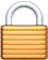to make a change in System Preferences, your Mac prompts you to approve with Apple Watch:To automatically enter your password without having to type it, just double-click the side button on your Apple Watch:## How to set up Auto Unlock

1. Make sure that your Mac and Apple Watch meet the system requirements for Auto Unlock.
2. Make sure that your devices are set up as follows:
• Your Mac has Wi-Fi and Bluetooth turned on.
• Your Mac and Apple Watch are signed in to iCloud with the same Apple ID, and your Apple ID is using two-factor authentication.
• Your Apple Watch is using a passcode.
3. Choose Apple menu  > System Preferences, then click Security & Privacy. Select "Use your Apple Watch to unlock apps and your Mac" or "Allow your Apple Watch to unlock your Mac."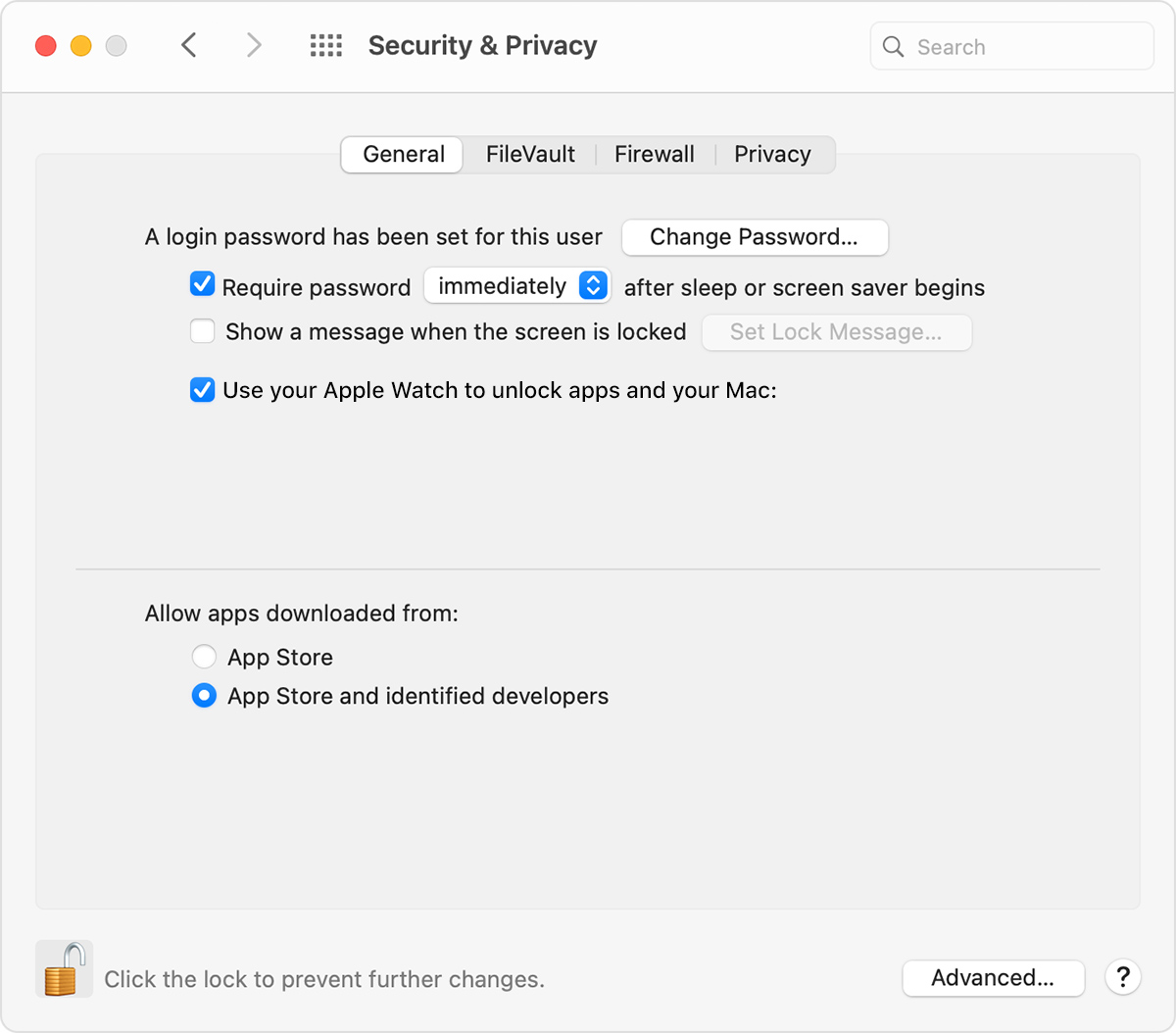If you're not sure whether your Mac supports Auto Unlock, press and hold the Option key while choosing Apple menu > System Information. Select Wi-Fi in the sidebar, then look for "Auto Unlock: Supported" on the right: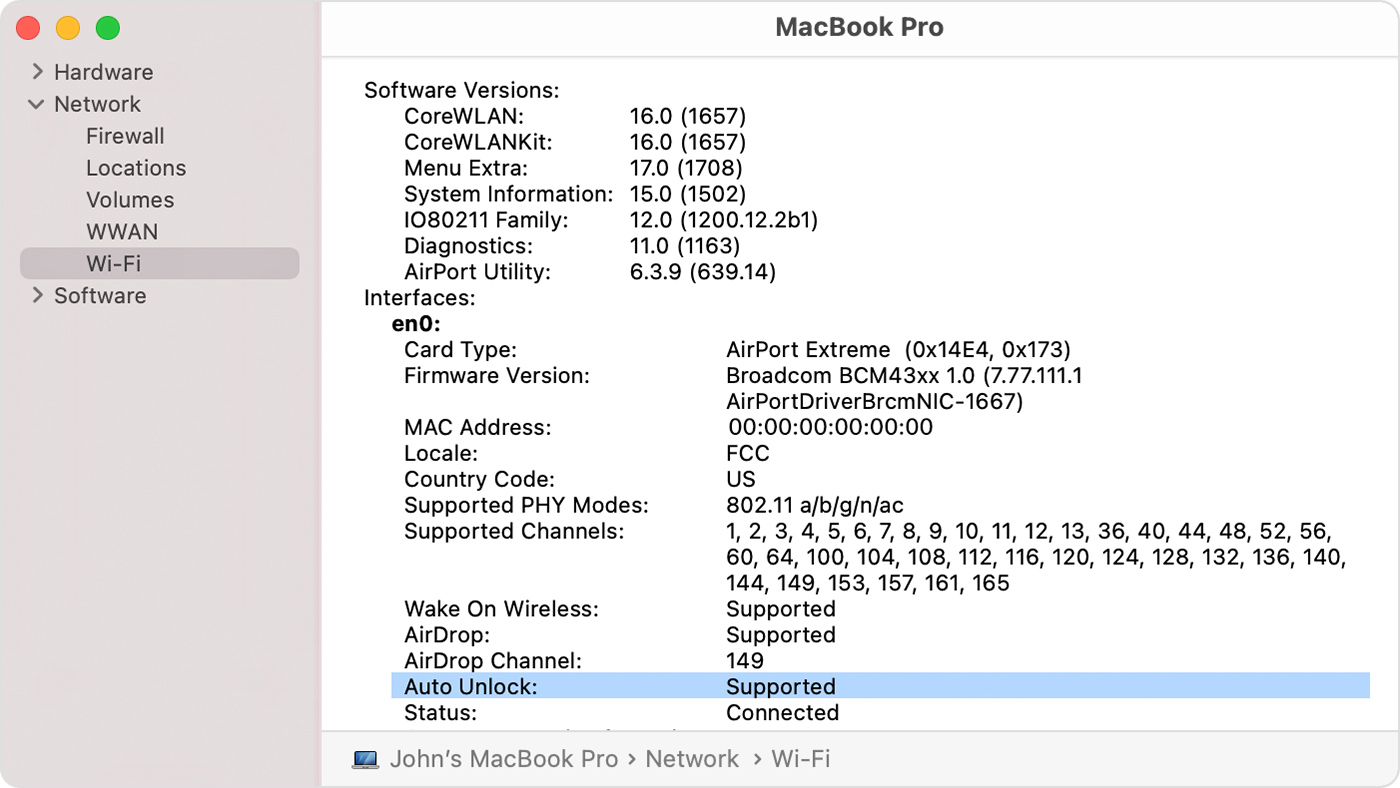If you're having difficulty getting Auto Unlock to work, try these solutions:

• In Security & Privacy preferences, deselect "Use your Apple Watch to unlock apps and your Mac," then restart your Mac and turn this setting back on.
• Make sure that your Mac isn't using Internet sharing or screen sharing.
Published Date:

# ERFC

The ERFC function returns the complementary Gauss error function of a value.

### Sample Usage

`ERFC(2)`

`ERFC(A2)`

### Syntax

`ERFC(z)`

• `z` - The number for which to calculate the complementary Gauss error function.

# COUNTUNIQUE

Counts the number of unique values in a list of specified values and ranges.

### Sample Usage

`COUNTUNIQUE(A1:C100)`

`COUNTUNIQUE(1,1,2,3,5,8,13,A2,B6:B9)`

### Syntax

`COUNTUNIQUE(value1, [value2, ...])`

• `value1` - The first value or range to consider for uniqueness.

• `value2, ...` - [ OPTIONAL ] - Additional values or ranges to consider for uniqueness.

### Notes

• Although `COUNTUNIQUE` is specified as taking a maximum of 30 arguments, Google Sheets supports an arbitrary number of arguments for this function.

`DCOUNTA`: Counts values, including text, selected from a database table-like array or range using a SQL-like query.

`DCOUNT`: Counts numeric values selected from a database table-like array or range using a SQL-like query.

`COUNTIF`: Returns a conditional count across a range.

Returns the number of values in a dataset.

`COUNTBLANK`: Returns the number of empty cells in a given range.

Returns the number of numeric values in a dataset.

# COUNTBLANK

Returns the number of empty cells in a given range.

### Sample Usage

`COUNTBLANK(A2:C100)`

### Syntax

`COUNTBLANK(value1, [value2,...])`

• value1 - The first value or range in which to count the number of blanks.
• value2 - [OPTIONAL ] - Additional values or ranges in which to count the number of blanks.

`DCOUNTA`: Counts values, including text, selected from a database table-like array or range using a SQL-like query.

`DCOUNT`: Counts numeric values selected from a database table-like array or range using a SQL-like query.

`COUNTUNIQUE`: Counts the number of unique values in a list of specified values and ranges.

`COUNTIF`: Returns a conditional count across a range.

`COUNTA`: Returns the number of values in a dataset.

`COUNT`: Returns the number of numeric values in a dataset.

# COMBIN

The COMBIN function returns the number of ways to choose some number of objects from a pool of a given size of objects.

### Sample Usage

`COMBIN(4,2)`

`COMBIN(A2,B2)`

### Syntax

`COMBIN(n, k)`

• `n` - The size of the pool of objects to choose from.

• `k` - The number of objects to choose.

### Notes

• `COMBIN` is the standard combinatorics function typically notated nCk and read "n choose k". This is equivalent to `FACT(n)/(FACT(k)*FACT(n-k))`.

`MULTINOMIAL`: Returns the factorial of the sum of values divided by the product of the values' factorials.

`FACTDOUBLE`: Returns the "double factorial" of a number.

`FACT`: The FACT function returns the factorial of a number.

### Examples

Mail might say that it can't connect to your email account, and repeatedly ask you to enter the password for it.

If your Mac is connected to the Internet but suddenly starts asking for your password, you should generally try again later, and if necessary work with your email provider to resolve. If you're using Apple's iCloud Mail, learn how to resolve issues with iCloud Mail.

Depending on the type of email account you're using, you might be able to correct the password as follows:

1. Quit Mail. If it doesn't quit, you can force it to quit.
2. Choose Apple menu  > System Preferences, then click Internet Accounts.
3. Select your mail account in the sidebar.
5. Close System Preferences, then open Mail and try using your account again.

## Test using webmail

Many email providers offer webmail, which is a way to use email with a web browser such as Safari, instead of with an email app such as Mail. For example, if you're using an iCloud Mail account, you can use iCloud.com to send and receive email.

Because the password that you use with Mail is usually the same password that you use with webmail, try signing in to webmail with that password. If it doesn't work there either, your password is probably incorrect. Your email provider can help you verify your email settings and make sure that you're using the correct password for the Mail app.

If no other solutions work, try setting up your email account again. To do that, first remove your email account, then add the account back.